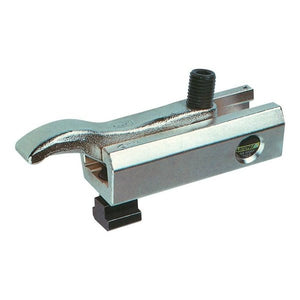## Categories# Lenzke Clamp MQ60 (to 80kN)

‚Ä¢ Strong Clamping pressure for small clamping torque
‚Ä¢ Flexible and adjustable at every angle to the T slot
‚Ä¢ Reduction of set-up time through reliable, fast and safe clamping
‚Ä¢ Vertically highly adjustable through triple clamping shoe
‚Ä¢ Widely adjustable clamping heights through stackable clamping shoe

M12 14 5-34mm V=38, L=75, L1=12, L2=20, B=52, b=24, h=37, kN=40 060-034-1214 147.56 - +
M12 14 22-45mm V=34, L=75, L1=12, L2=20, B=52, b=24, h=37, kN=40 060-045-1214 126.49 - +
M12 14 5-40mm V=58, L=115, L1=12, L2=28, B=52, b=24, h=37, kN=40 060-040-1214 173.45 - +
M12 14 35-67mm V=58, L=115, L1=12, L2=28, B=52, b=24, h=37, kN=40 060-067-1214 168.00 - +
M16 18 6-38mm V=45, L=85, L1=15, L2=25, B=60, b=28, h=42, kN=55 060-038-1618 999.00 - +
M16 18 6-58mm V=80, L=130, L1=15, L2=32, B=60, b=28, h=42, kN=55 060-058-1618 176.53 - +
M20 22 5-35mm V=49, L=100, L1=19, L2=32, B=75, b=34, h=48, kN=65 060-035-2022 241.85 - +
M20 22 28-55mm V=49, L=100, L1=19, L2=32, B=75, b=34, h=48, kN=65 060-055-2022 194.74 - +
M20 22 8-68mm V=88, L=150, L1=19, L2=38, B=75, b=34, h=48, kN=65 060-068-2022 265.00 - +
M20 22 45-85mm V=78, L=150, L1=19, L2=38, B=75, b=34, h=48, kN=65 060-085-2022 275.84 - +
M24 28 10-46mm V=66, L=125, L1=22, L2=38, B=88, b=42, h=57, kN=80 060-046-2428 289.96 - +
M24 28 15-80mm V=105, L=180, L1=22, L2=45, B=88, b=42, h=57, kN=80 060-082-2428 999.00 - +
M30 36 10-46mm V=69, L=125, L1=25, L2=45, B=88, b=42, h=57, kN=80 060-046-3036 298.73 - +
M30 36 15-80mm V=108, L=180, L1=25, L2=45, B=88, b=42, h=57, kN=80 060-080-3036 999.00 - +